Transforming Numerical Methods Education for the STEM Undergraduate MOBILE | VIDEOS | BLOG | YOUTUBE | TWITTER | COMMENTS | ANALYTICS | ABOUT | CONTACT | COURSE WEBSITES | BOOKS | MATH FOR COLLEGE |EXPERIMENTS FOR NUMERICAL METHODS   Low cost, low space, low setup time experiments have been developed for a course in numerical methods.  Read the following paper to find out how the experiments are being implemented and assessed in the classroom. The Length of a Curve   This experiment illustrates the difference in using curve fitting with polynomial interpolants and spline interpolants.    To the student: A handout is given to the students that gives the background information on the experiment, how to collect the data, and exercises that need to solved.   To the Instructor: Do you want to put this experiment together for your class? Find out to see what materials you need to buy, where you can buy the materials, and how much it costs.   Experimental Data:  If you do not want to conduct the experiment but want the data.   Topics Covered: Interpolation and Integration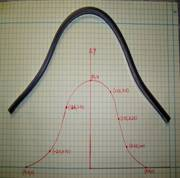Strains in a Loaded Truss   This experiment illustrates the use of simultaneous linear equations in finding the forces in the members of a truss.    To the student: A handout is given to the students that gives the background information on the experiment, how to collect the data, and exercises that need to solved.   Experimental Data:  If you do not want to conduct the experiment but want the data.   Topics Covered: Simultaneous Linear Equations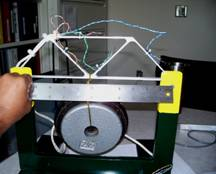Cooling a Aluminum Cylinder in Iced Water   This experiment illustrates several key concepts of numerical methods, mainly regression to find physical constants from the collected data.    To the student: A handout is given to the students that gives the background information on the experiment, how to collect the data, and exercises that need to solved.   Experimental Data:  If you do not want to conduct the experiment but want the temperature vs time data.  You will need to look at the handout also to get other needed data.   Topics Covered: Regression, Nonlinear Equations, Integration, Ordinary Differential Equations, Differentiation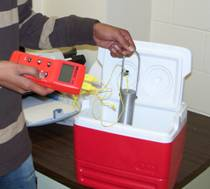Twisting a Mousetrap Spring This experiment illustrates the concept of regression to find the constants of a linear model of a torsional spring.  Integration is used to find the torsional energy stored in the spring. 'To the student: A handout is given to the students that gives the background information on the experiment, how to collect the data, and exercises that need to solved.   Experimental Data:  If you do not want to conduct the experiment but want the forces vs angle data.  Topics Covered: Regression, Integration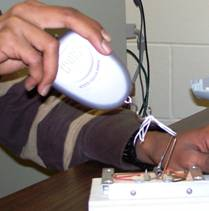Hold Your Water in a Champagne Glass   This experiment illustrates several key concepts of numerical methods, mainly spline interpolation and integration.    To the student: A handout is given to the students that gives the background information on the experiment, how to collect the data, and exercises that need to solved.   To the Instructor: Do you want to put this experiment together for your class? Find out to see what materials you need to buy, where you can buy the materials, and how much it costs.   Experimental Data:  If you do not want to conduct the experiment but want the radius vs height data.    Topics Covered: Interpolation, Integration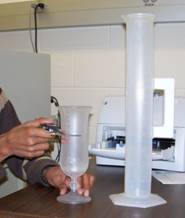Where is the center - the center of gravity   The motivation behind this experiment is to understand spline interpolation and integration to find the center of mass of a thin plate of arbitrary shape   To the student: A handout is given to the students that gives the background information on the experiment, how to collect the data, and exercises that need to solved.   To the Instructor: Do you want to put this experiment together for your class? Find out to see what materials you need to buy, where you can buy the materials, and how much it costs.   Experimental Data:  If you do not want to conduct the experiment but want the height vs length data.    Topics Covered: Interpolation, Integration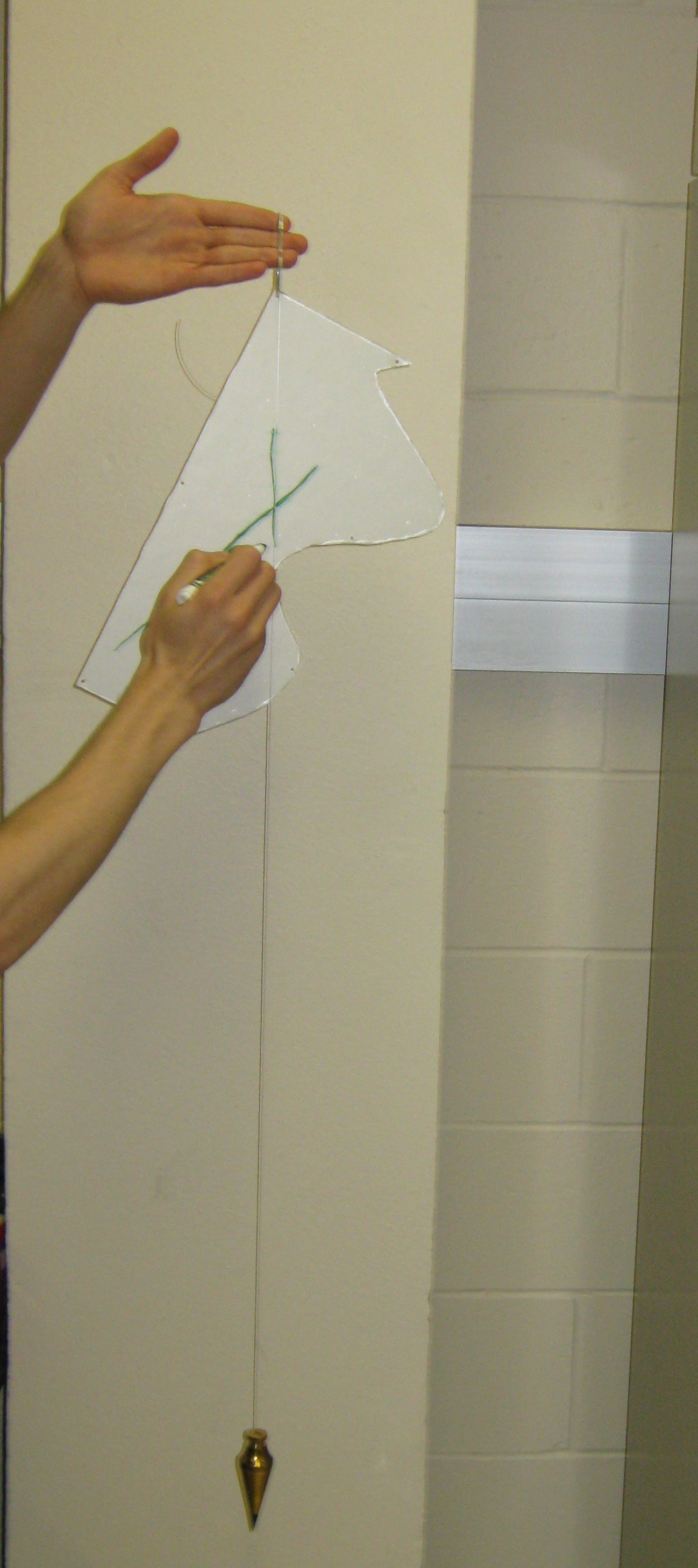AUDIENCE |  AWARDS  |  PEOPLE  |  TRACKS  |  DISSEMINATION  |  PUBLICATIONS Copyrights: University of South Florida, 4202 E Fowler Ave, Tampa, FL 33620-5350. All Rights Reserved. Questions, suggestions or comments, contact kaw@eng.usf.edu  This material is based upon work supported by the National Science Foundation under Grant#0126793, 0341468, 0717624,  0836981, , 0836805.  Any opinions, findings, and conclusions or recommendations expressed in this material are those of the author(s) and do not necessarily reflect the views of the National Science Foundation.  Other sponsors include Maple, MathCAD, USF, FAMU and MSOE.  Based on a work at http://mathforcollege.com/nm.  Holistic Numerical Methods licensed under a Creative Commons Attribution-NonCommercial-NoDerivs 3.0 Unported License. ANALYTICS